Question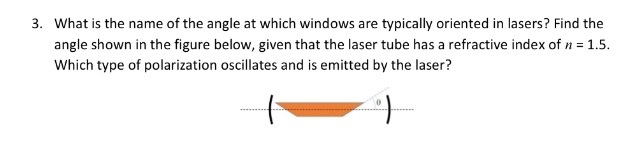The angle at which windows are typically oriented in lasers is called Brewster's angle.

It is an angle of incidence at which p- polarized light is perfectly transmitted through a transparent dielectric surface.

at this angle, the unpolarized (monochromatic or light with various wavelengths ) is incident, light is s- polarized and reflected.

glass refraction index= 1.5 , and refraction index of air = 1.

therefore , Brewster’s angle = tan-1(1.5/1) = 56.3o

polarization is of two types P- polarized(electric field parallel to the plane of the incident) and s- polarization(electric field perpendicular to plane of the incident)

when the light is incident at this angle, then it is reflected and produce s-polarized.

when p- polarized light is incident, then it is perfectly transmitted without reflection

#### Earn Coins

Coins can be redeemed for fabulous gifts.

Similar Homework Help Questions
• ### (a) The optical gain coefficient of a semiconductor laser with 150 μm cavity length is shown in Fig 1. The loss coefficient and the refractive index of the semiconductor laser are 21.6 cm" and 3....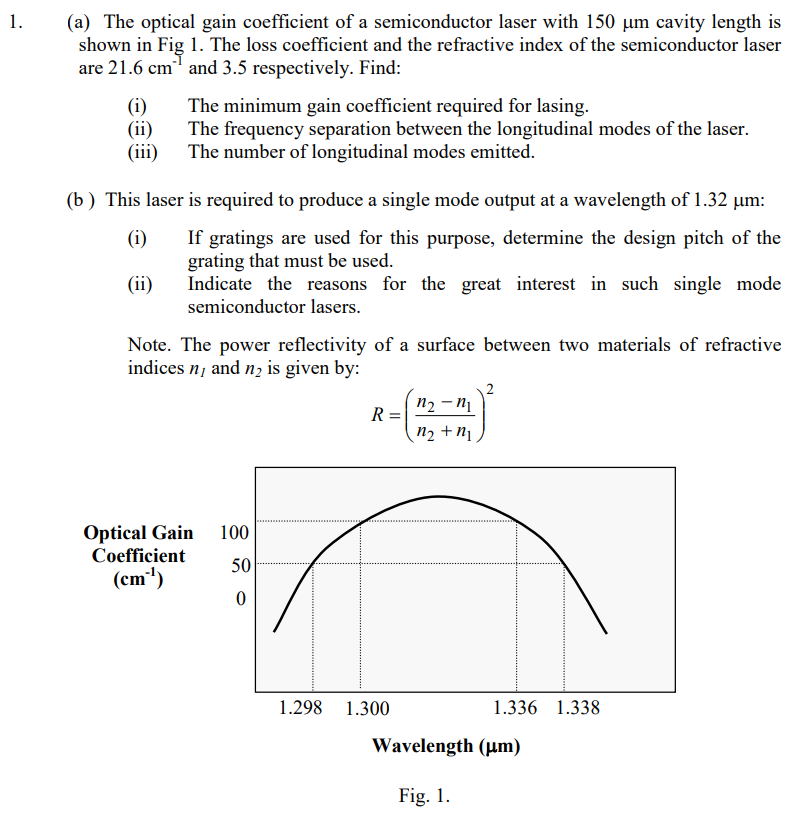(a) The optical gain coefficient of a semiconductor laser with 150 μm cavity length is shown in Fig 1. The loss coefficient and the refractive index of the semiconductor laser are 21.6 cm" and 3.5 respectively. Find (i)The minimum gain coefficient required for lasing (ii) The frequency separation between the longitudinal modes of the laser (iii) The number of longitudinal modes emitted (b) This laser is required to produce a single mode output at a wavelength of 1.32 unm If...

• ### (c) A laser diode operating at 1,064nm has an internal cavity loss (a) of 200 m...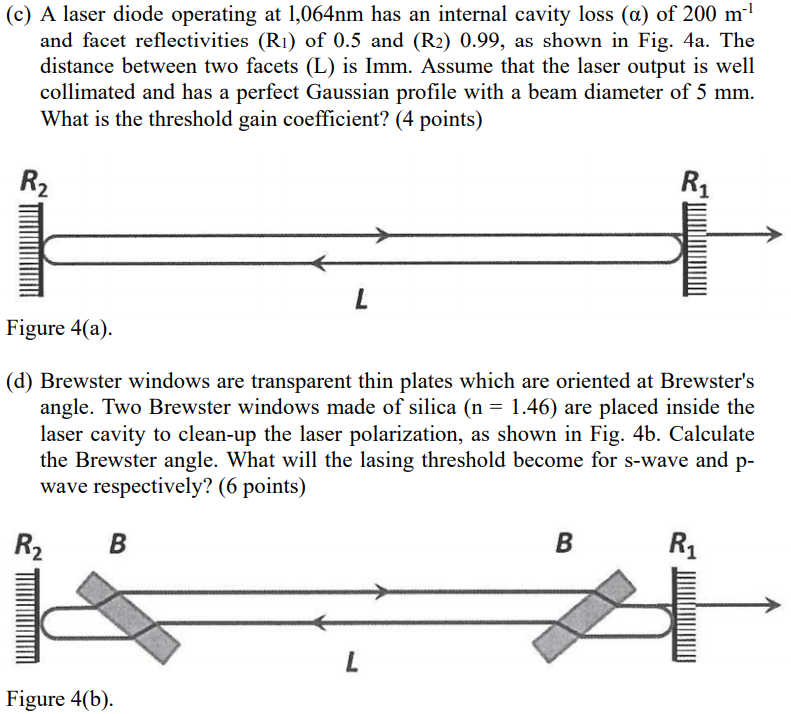(c) A laser diode operating at 1,064nm has an internal cavity loss (a) of 200 m and facet reflectivities (R1) of 0.5 and (R2) 0.99, as shown in Fig. 4a. The distance between two facets (L) is Imm. Assume that the laser output is well collimated and has a perfect Gaussian profile with a beam diameter of 5 mm. What is the threshold gain coefficient? (4 points) R₂ R1 L Figure 4(a). (d) Brewster windows are transparent thin plates which...

• ### 2. Lasers (20 points) A He-Ne laser operating atA-632.8 nm produces 50 mW of multimode power...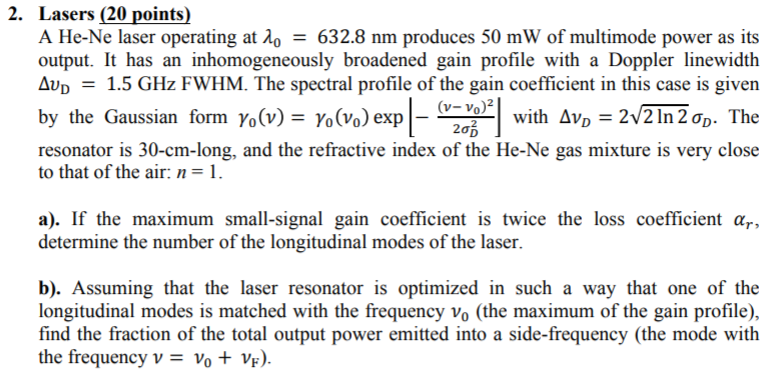2. Lasers (20 points) A He-Ne laser operating atA-632.8 nm produces 50 mW of multimode power as its output. It has an inhomogeneously broadened gain profile with a Doppler linewidth Av1.5 GHz FWHM. The spectral profile of the gain coefficient in this case is given (V-Vo)2 y the Gaussian form Yo(v)-Yo(Vo) exp | WIth ΔνD_ ー resonator is 30-cm-long, and the refractive index of the He-Ne gas mixture is very close to that of the air: n-1 a). If the...

• ### Problem 9 145 What is the angle of refraction in the prism in degrees? Given a...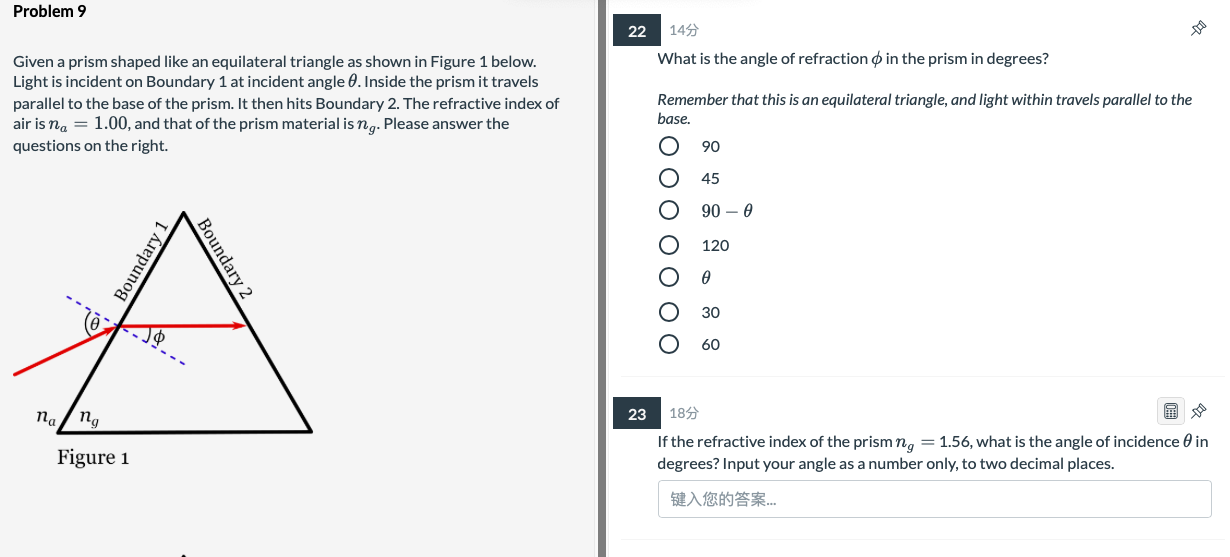Problem 9 145 What is the angle of refraction in the prism in degrees? Given a prism shaped like an equilateral triangle as shown in Figure 1 below. Light is incident on Boundary 1 at incident angle 8. Inside the prism it travels parallel to the base of the prism. It then hits Boundary 2. The refractive index of air is na = 1.00, and that of the prism material is ng. Please answer the questions on the right. Remember...

• ### (a) Briefly explain what is meant by the following terms: refraction, refractive index, angle of incidence,...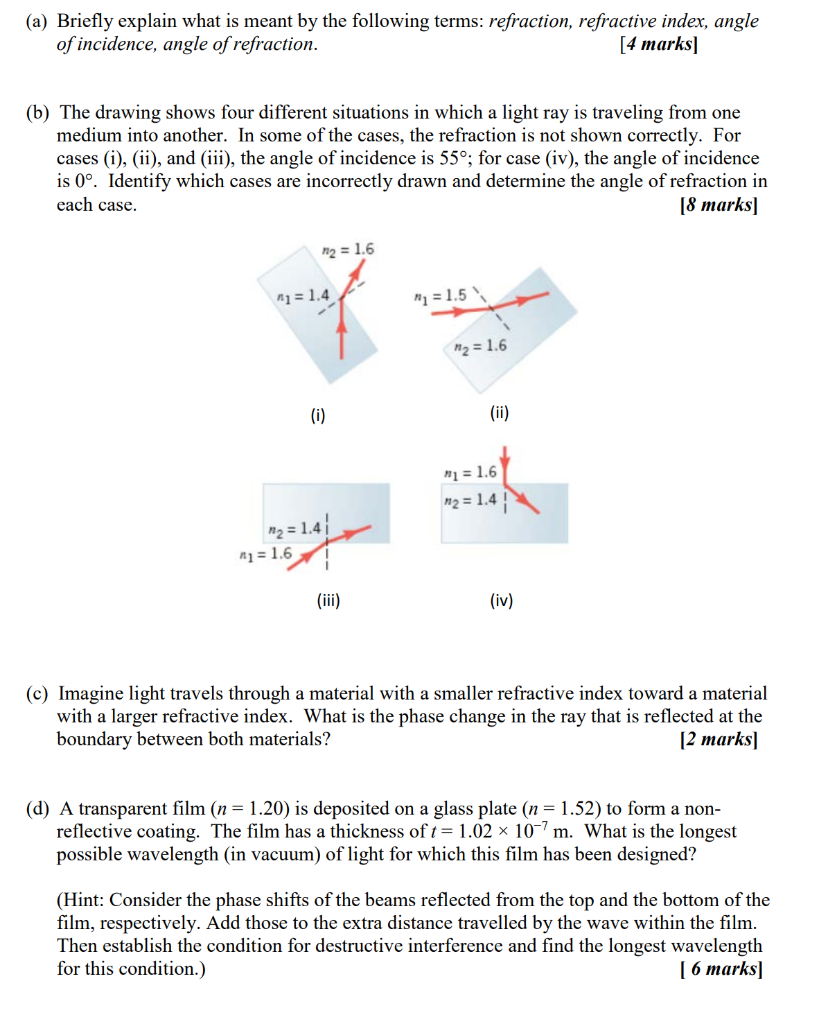(a) Briefly explain what is meant by the following terms: refraction, refractive index, angle of incidence, angle of refraction. [4 marks] (b) The drawing shows four different situations in which a light ray is traveling from one medium into another. In some of the cases, the refraction is not shown correctly. For cases (i), (ii), and (iii), the angle of incidence is 55°; for case (iv), the angle of incidence is 0°. Identify which cases are incorrectly drawn and determine...

• ### asap Question 16 of 31 For the optical fiber shown in the figure, find the minimum...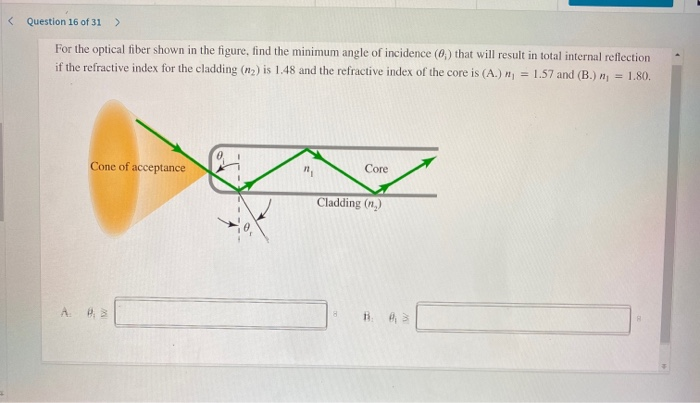asap Question 16 of 31 For the optical fiber shown in the figure, find the minimum angle of incidence (0) that will result in total internal reflection if the refractive index for the cladding (n) is 1.48 and the refractive index of the core is (A.) n = 1.57 and (B.) n = 1.80. Cone of acceptance Core Cladding (n.) A 8 1:42 Question 15 of 31 > Building contractors often install double-glazed windows to prevent thermal energy (heat) from...

• ### Consider a fiber from which the cladding is removed over a short length as shown in figure below. Assume that the refractive indices of core and cladding are 1.5 and 1.4, respectively. What will happen to the output power if the bare portion of the fiber

Consider a fiber from which the cladding is removed over a short length as shown in figure below. Assume that the refractive indices of core and cladding are 1.5 and 1.4, respectively. What will happen to the output power if the bare portion of the fiber is covered by a liquid whose refractive index is varied from 1.4 to 1.5?

• ### 1. KDP A typical electro-optic amplitude modulator is shown above. A linearly polarized beam with wavelength 2 633nm is sent a KDP crystal which is oriented such that when a voltage is applied across...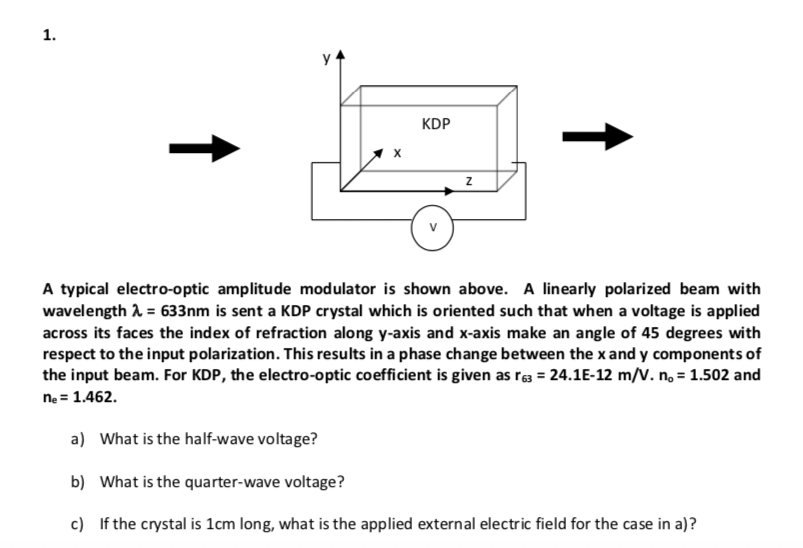1. KDP A typical electro-optic amplitude modulator is shown above. A linearly polarized beam with wavelength 2 633nm is sent a KDP crystal which is oriented such that when a voltage is applied across its faces the index of refraction along y-axis and x-axis make an angle of 45 degrees with respect to the input polarization. This results in a phase change between the x and y components of the input beam. For KDP, the electro-optic coefficient is given as...

• ### 1. KDP A typical electro-optic amplitude modulator is shown above. A linearly polarized beam with wavelength 2 633nm is sent a KDP crystal which is oriented such that when a voltage is applied across...1. KDP A typical electro-optic amplitude modulator is shown above. A linearly polarized beam with wavelength 2 633nm is sent a KDP crystal which is oriented such that when a voltage is applied across its faces the index of refraction along y-axis and x-axis make an angle of 45 degrees with respect to the input polarization. This results in a phase change between the x and y components of the input beam. For KDP, the electro-optic coefficient is given as...

• ### 1. KDP A typical electro-optic amplitude modulator is shown above. A linearly polarized beam with wavelength 633nm is sent a KDP crystal which is oriented such that when a voltage is applied across i...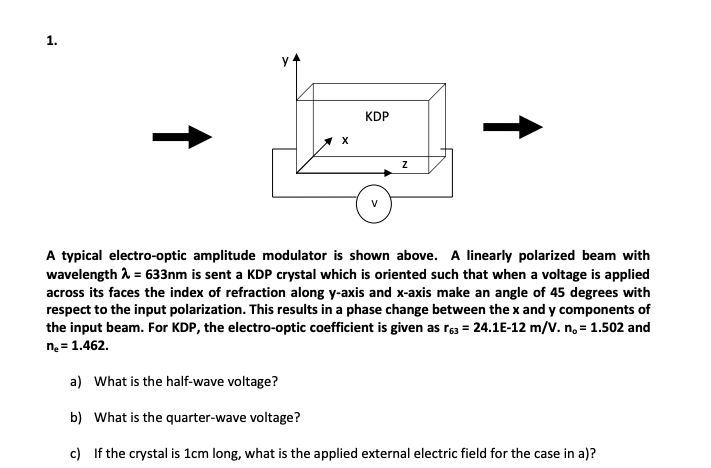1. KDP A typical electro-optic amplitude modulator is shown above. A linearly polarized beam with wavelength 633nm is sent a KDP crystal which is oriented such that when a voltage is applied across its faces the index of refraction along y-axis and x-axis make an angle of 45 degrees with respect to the input polarization. This results in a phase change between the x and y components of the input beam. For KDP, the electro-optic coefficient is given as r6...